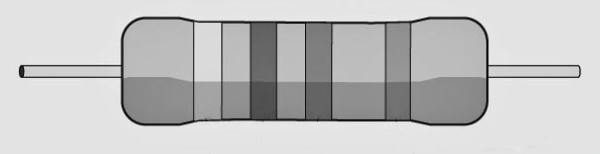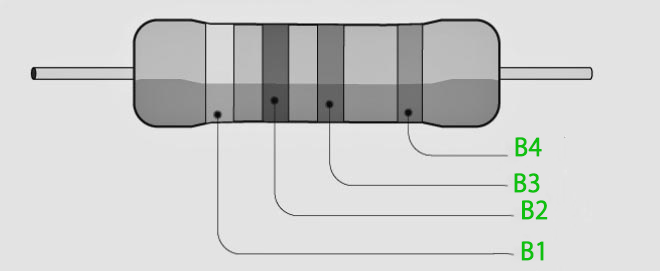GeeksforGeeks App
Open AppBrowser
Continue

# Colour code for Carbon Resistors

Many inventions and discoveries have been produced to make life easier for humans. One such discovery that we are extremely reliant on to make our lives easier is the discovery of current electricity. The discovery of electricity is credited to Benjamin Franklin.

The flow of electrons from one segment of the circuit to another is referred to as current electricity. A pace of flowing electric charge past a place or region is referred to as an electric current. When there is a net movement of electric charge through a region, an electric current exists. Charge carriers are moving particles, and different sorts of particles can be found in different conductors. Electrons flowing through a wire are the charge carriers in electric circuits. Ions are charged carriers in an electrolyte, while ions and electrons are charge carriers in an ionised gas. An ammeter is a device used to measure electric current. In incandescent light bulbs, electric current induces Joule heating, which produces light.

When an electric current flows through a bulb or any other conductor, the conductor creates an obstacle, which is known as electrical resistance.

### What is Electrical Resistance?

The electrical resistance of a circuit is the ratio between the voltage applied to the current flowing through it. There is a relationship between the current running through a conductor and the potential difference across it, according to Ohm’s law. It is provided by

V ∝ I V = IR

or

R = V/I

where,

• V is the potential difference across the conductor (Volts)
• I is the current through the conductor (Amperes)
• R is the constant of proportionality called resistance (in ohms)

### Resistors

A Resistor is a device used for restricting the flow of electric current in the circuit. Some of the uses of resistors are heating, LEDs and transistor, dividing voltage, frequency and timing, circuit functions.

Resistor definition explains that it is a basic component found in all electronic gadgets. The resistor is a two-terminal passive electrical component. The resistor is used to limit the passage of electric current in a circuit, according to its definition. One of the most frequent forms of electronics is the carbon resistor. They’re comprised of a solid cylindrical resistive element with wire leads or metal end caps implanted inside.

The SI unit of the resistor is Ohm (Ω).

There are two basic types of resistors as follows:

• Linear resistor: Linear resistors are resistors whose values fluctuate as the applied temperature and voltage change.
• Fixed resistors: These resistors have a predetermined value that cannot be altered. The many types of fixed resistors are as follows:
• Carbon composition resistors
• Wire wound resistors
• Thin-film resistors
• Thick film resistors
• Variable resistors: These resistors do not have a fixed value and may be adjusted with the use of a dial, knob, or screw. These resistors are used to adjust the volume and tone of radio receivers. The many types of variable resistors are as follows:
• Potentiometers
• Rheostats
• Trimmers
• Non-linear resistor – The resistor values change with temperature and applied voltage and are not governed by Ohm’s law. The various varieties of non-linear resistors are as follows:
• Thermistors
• Varistors
• Photoresistors

### What are Carbon Resistors?

Materials like nichrome, brass, platinum, and tungsten, which are metal, and alloys are used to produce the resistance. However, most of these metals have low electrical resistance compared to carbon resistors, as it makes it difficult to produce high resistance without making the resistor bulky.

Resistance ∝  (Length × Resistivity)Carbon Resistor

Carbon resistors are favoured for the vast majority of applications. This is due to the fact that they are inexpensive to manufacture, small, and can be directly printed onto circuit boards (like the computer processors in phones and tablets). Within practical constraints, they also reproduce resistance fairly well. In comparison to metal wires, which are costly to create, carbon is abundant and inexpensive.

Carbon resistors come in different physical sizes with power dissipation limits commonly from 1 W to 1/8 W. They are made from a solid cylindrical resistive element with embedded wire leads or metal end caps and consist of a ceramic core, on which a thin layer of crystalline carbon is deposited. There is only one disadvantage of Carbon resistors i.e they cannot withstand very temperature (with an increase in temperature there is a change in the resistivity).

Parts of a Carbon Resistor

Resistors can be made from a wide range of materials. The many components of a carbon resistor are as follows:

• Ceramic Core
• Nickel Cap
• Carbon Film
• Protective Lacquer

The deposition procedure is used to make the carbon resistors. The carbon layer is deposited on the resistor’s ceramic body in this method. Because the carbon film slows current flow, it is an essential component of the resistor. Hydrocarbons such as methane and benzene are shattered at a high temperature of 1000 °C. Pure graphite (carbon) is used for distribution on the ceramic body, and the ceramic mould functions as an insulator against high temperatures or electricity. Because of the carbon covering, the resistor is able to endure electricity without being damaged.

Uses of Carbon Resistors:

• Carbon is deposited onto a ceramic core in the carbon resistor. The deposited carbon is etched in a spiral, which transforms it into a wire wound on a ceramic core.
• The Pitch, diameter, and length of the carbon spiral vary depending on the resistance required. Nickel caps are added to both ends of the core to ensure that the carbon and lead make good contact. For electrical insulation, the leads are soldered to the nickel caps, and the entire resistor is coated with lacquer.
• Metal caps operate as heat sinks for small resistances necessary for small currents, carrying away heat dissipated by the resistor. A metal heat sink is added separately for larger current requirements to carry away excess heat and prevent the resistor from burning up.
• To indicate the resistance, carbon resistors are colour-coded.

How Does Carbon Resistor Work?

When building an electric or electronic circuit for a certain purpose, the exact amount of resistance in the circuit board must be induced. It is accomplished by using carbon resistors, which are small components. These components are a small packet of resistance that is plugged into the circuit to decrease the flow of current by a specified amount.

• The Carbon resistor’s ability to endure high energy pulses is one of its most significant advantages.
• When current travels through a carbon resistor, the entire body conducts the energy.
• Carbon resistors are less expensive to manufacture and have a higher resistance than wire-wound resistors.
• A major disadvantage of a carbon resistor is its large negative coefficient of resistance, which causes the resistance to fluctuating rapidly when the temperature changes. The resistance of the resistor reduces as the temperature rises.

### What is the Resistor colour code?

Because of their small size, it is challenging to print resistance values on the resistors. To solve this problem the resistor colour code was invented in the 1920s by the Radio Manufacturers Association (RMA).

Colour bands are used to identify any leaded resistors with a power rating of less than one watt. They are specified by a number of bands, which collectively determine the resistance value, tolerance rate, and, in some cases, dependability and failure rates. A resistor’s number of bands might range from three to six. The resistance value is indicated by the first two bands, while the third band acts as a multiplier. Let’s look at how to read resistor colour codes, look at an example, and learn a mnemonic to remember the number sequence in this post.

### Resistor Color Code Table

To memorize this table one can use B.B. ROY of Great Britain had a Very Good Wife.

### How to read Resistor Colour Code?

To read get the resistance of the resistor hold the resistor in such a way that the bands are clearly visible. There are four bands from which we can calculate the resistance.

• B1 – Indicates First significant figure
• B2 – Indicates Second significant figure
• B3 – Indicates multiple of 10
• B4 – Shows tolerance ( % error in the result)

The fourth band is generally placed a little further away from the other bands.Resistor Colour Code

For example, If there is a Question to find the value of carbon resistor with colour bands Red, Red, Red, Silver.

B1 – Red  2

B2 – Red 2

B3 – Red 102

B4 –  Silver +-10%

So, the resistance will be 22 x 102 +- 10% Ω

### Sample Questions

Question 1: What is the Symbol of Carbon Resistor?

The most commonly used symbol for a resistor in electrical and electronic circuit designs is a zig-zag line, with the value of its resistance expressed by the Ohms. Fixed resistors typically have only one resistance value. Variable resistors, on the other hand, can have an endless number of resistances ranging from zero to their maximum value.

Question 2: What is a resistor? What is the SI unit of resistor?

A Resistor is a device used for restricting the flow of electric current in the circuit. The SI unit of resistor is Ohm(Ω).

Question 3: Why do Carbon Resistors have coloured bands on them?

Because resistors are normally very small in size which makes it difficult to print resistor values on them. So, colour bands are printed on them to give the value of their electrical resistance.

Question 4: Which is the easiest way to remember resistor colour code?

The resistor colour codes can be remembered with the help of a phrase “BB ROY of Great Britain had a Very Good Wife,” the capital letters in this phrase represent the first letters of the colours and their positions. For example the First Letter “B” stands for Black, Second “B” stands for Brown.

Question 5: When was the Resistor Colour Code invented?

The resistor colour code was developed in the 1920’s by the Radio Manufacturers Association (RMA).

Question 6: Find the value of carbon resistor with colour bands Yellow, Violet, Brown, Gold.

Yellow – 4

Violet – 7

Brown – 101

Gold –  +-5%

Resistivity = 47 X 101 +- 5% Ω

Question 7: Find the value of carbon resistor with colour bands Red, Yellow, Gold.

Red – 2

Yellow – 4

Gold – 10-1

No Colour – +-20%

Resistivity = 24 X 10-1 +- 20% Ω

My Personal Notes arrow_drop_up
Related Tutorials